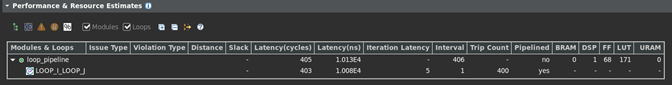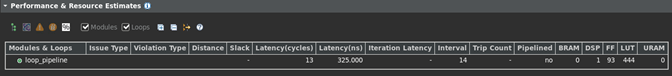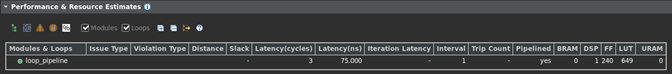# Working with Nested Loops - 2022.2 English

## Vitis High-Level Synthesis User Guide (UG1399)

Document ID
UG1399
Release Date
2022-12-07
Version
2022.2 English

To get the best performance (lowest latency) when working with nested loops, it becomes crucial to create perfectly nested loops. In a perfect nested loop, the loop bounds are constant and only the innermost loop contains any functionality (as shown below)

``````Perfect_nested_loop_1: for (int i = 0; i < N; ++i) {
Perfect_nested_loop_2: for (int j = 0; j < M; ++j) {
// Perfect Nested Loop Code goes here and no where else
}
}

Imperfect_nested_loop_1: for (int i = 0; i < N; ++i) {
// Imperfect Nested Loop Code contains code here
Imperfect_nested_loop_2: for (int j = 0; j < M; ++j) {
// Imperfect Nested Loop Code goes here
}
// Imperfect Nested Loop Code may contain code here as well
}``````
Perfect loop nest
Only the innermost loop has loop body content, there is no logic specified between the loop statements and all the loop bounds are constant
Semi-perfect loop nest
Only the innermost loop has loop body content, there is no logic specified between the loop statements but the outermost loop bound can be a variable.
Imperfect loop nest
The inner loop has variable bounds or the loop body is not exclusively inside the inner loop. In this case designers should try to restructure the code or unroll the loops in the loop body to create a perfect loop nest.

It also requires additional clock cycles to move between rolled nested loops. It requires one clock cycle to move from an outer loop to an inner loop or from an inner loop to an outer loop. In the small example shown here, this implies 200 extra clock cycles to execute the loop `Outer`.

``````void foo_top { a, b, c, d} {
...
Outer: while(j<100)
Inner: while(i<6) // 1 cycle to enter inner
...
LOOP_BODY
...
} // 1 cycle to exit inner
}
...
}``````

The LOOP_FLATTEN pragma or directive is used to allow labeled perfect and semi-perfect nested loops to be flattened, removing the need to re-code for optimal hardware performance and reducing the number of cycles it takes to perform the operations in the loop. When the LOOP_FLATTEN optimization is applied to a set of nested loops, it should be applied to the innermost loop that contains the loop body. Loop flattening can also be performed either by applying it to individual loops or applying it to all loops in a function by applying the directive at the function level.

When pipelining nested loops, the optimal balance between area and performance is typically found by pipelining the innermost loop. This also results in the fastest runtime. The following code example, pipelined_loop available on GitHub, demonstrates the trade-offs when pipelining loops and functions.

``````#include "loop_pipeline.h"

dout_t loop_pipeline(din_t A[N]) {
int i,j;
static dout_t acc;

LOOP_I:for(i=0; i < 20; i++){
LOOP_J: for(j=0; j < 20; j++){
acc += A[j] * i;
}
}
return acc;
}``````

In the above example, if the innermost (`LOOP_J`) is pipelined, there is one copy of `LOOP_J` in hardware (a single multiplier). Vitis HLS automatically flattens the loops when possible, as in this case, and effectively creates a new single loop (now called `LOOP_I_LOOP_J`) with 20*20 iterations. Only one multiplier operation and one array access need to be scheduled, then the loop iterations can be scheduled as a single loop-body entity (20x20 loop iterations).

Tip: When a loop or function is pipelined, any loop in the hierarchy below the loop or function being pipelined must be unrolled.

If the outer loop (`LOOP_I`) is pipelined, inner-loop (`LOOP_J`) is unrolled creating 20 copies of the loop body: 20 multipliers and 1 array accesses must now be scheduled. Then each iteration of `LOOP_I` can be scheduled as a single entity.

If the top-level function is pipelined, both loops must be unrolled: 400 multipliers and 20 array accesses must now be scheduled. It is very unlikely that Vitis HLS will produce a design with 400 multiplications because, in most designs, data dependencies often prevent maximal parallelism, for example, even if a dual-port RAM is used for `A`, the design can only access two values of `A` in any clock cycle. Otherwise, the array must be partitioned into 400 registers, which then can all be read in one clock cycle, with a very significant HW cost.

The concept to appreciate when selecting at which level of the hierarchy to pipeline is to understand that pipelining the innermost loop gives the smallest hardware with generally acceptable throughput for most applications. Pipelining the upper levels of the hierarchy unrolls all sub-loops and can create many more operations to schedule (which could impact compile time and memory capacity), but typically gives the highest performance design in terms of throughput and latency. The data access bandwidth must be matched to the requirements of the operations that are expected to be executed in parallel. This implies that we might need to partition array A in order to make this work.

To summarize the above options:

• Pipeline `LOOP_J`: Latency is approximately 400 cycles (20x20) and requires less than 250 LUTs and registers (the I/O control and FSM are always present).

Figure 1. Performance & Resource Estimates• Pipeline `LOOP_I`: Latency is 13 cycles but requires a few hundred LUTs and registers. About twice the logic as the first option, minus any logic optimizations that can be made.

Figure 2. Performance & Resource Estimates• Pipeline function `loop_pipeline`: Latency is now only 3 cycles (due to 20 parallel register accesses) but requires almost twice the logic as the second option (and about 4 times the logic of the first option), minus any optimizations that can be made.

Figure 3. Performance & Resource EstimatesVitis HLS cannot flatten imperfect loop nests. This will result in additional clock cycles to enter and exit the loops. When the design contains nested loops, analyze the results to ensure that as many nested loops as possible have been flattened: review the log file or look in the synthesis report for cases (as shown above) where the loop labels have been merged (`LOOP_I` and `LOOP_J` are now reported as `LOOP_I_LOOP_J`).